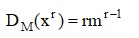# Theory of Value and Unknown Choice

#### Theory of Value and Unknown Choice :

Farmers and industrialists produce their products but can not sell it with known price. The price depends on the quality and demands. In mathematics and in the process of calculation of a derivative we use value and unknown choice. In the implicit derivative calculation, we must pay heed to the recognition of the independent variable from function variable if the X is independent variable, then Y is function of x, hence DxX=1 but Dxy is unknown.

Explanation: If the theory of value and unknown choice is the same for all economic activists in the global market, we will use it in Iranian school of economic thought as the law of value and unknown choice.

Other implicit derivative calculation: Dx M=1 but Dxy is unknown the same as0 replies